# Laws of Indices'Indices' is the plural form of 'index'. And, index means the power of any number or variable term, which is called a base. Power or index is also known as an exponent.

********************

10 Math Problems officially announces the release of Quick Math Solver, an Android App on the Google Play Store for students around the world.

********************There are certain rules which are used to solve the problems of indices. These rules are called the laws of indices. Some laws of indices are as follows:## Laws of Indices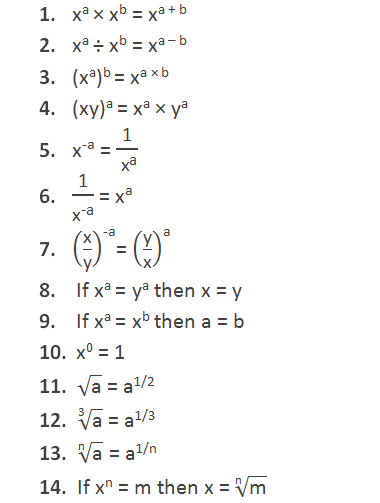### Problems involving laws of indices

Example 1: Find the value of: 274/3

Solution:

Here,Example 2: Find the value of: (2401)-1/4

Solution:

Here,Example 3: Find the value of:Solution:

Here,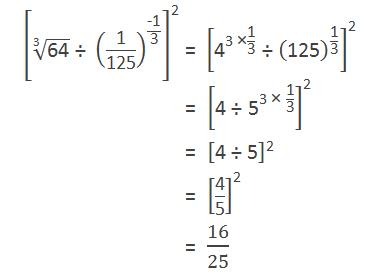Example 4: Find the value of:Solution:

Here,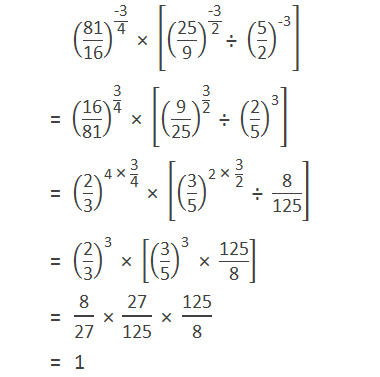Example 5: Find the value of: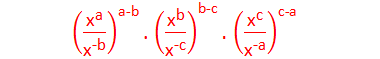Solution:

Here,Example 6: Simplify: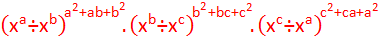Solution:

Here,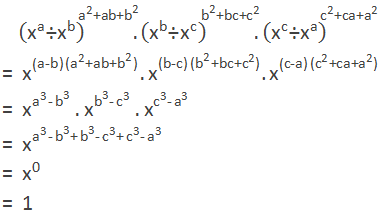Example 7: Simplify:Solution:

Here,Example 8: Simplify:Solution:

Here,Example 9: Simplify:Solution:

Here,Example 10: Find the value of:Solution:

Here,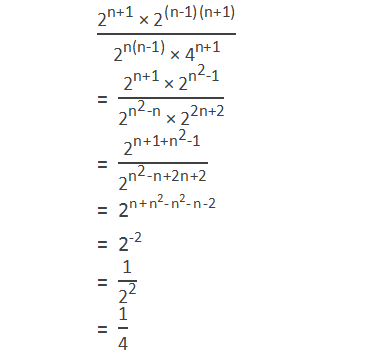If you have any question or problems regarding the law’s  of indices, you can ask here, in the comment section below.

1.X to the power y equal to y to the power x .x equal twice y .

2.This comment has been removed by the author.

3.Subsequently, to help you in tracking down the best criminal lawyer, examining the different areas and classes of criminal law is fundamental.
stephenjackcriminallawyer

4.Corporate mergers and acquisitions are subject to stringent legal scrutiny to ensure fair competition and market efficiency. Yesfinancialfree